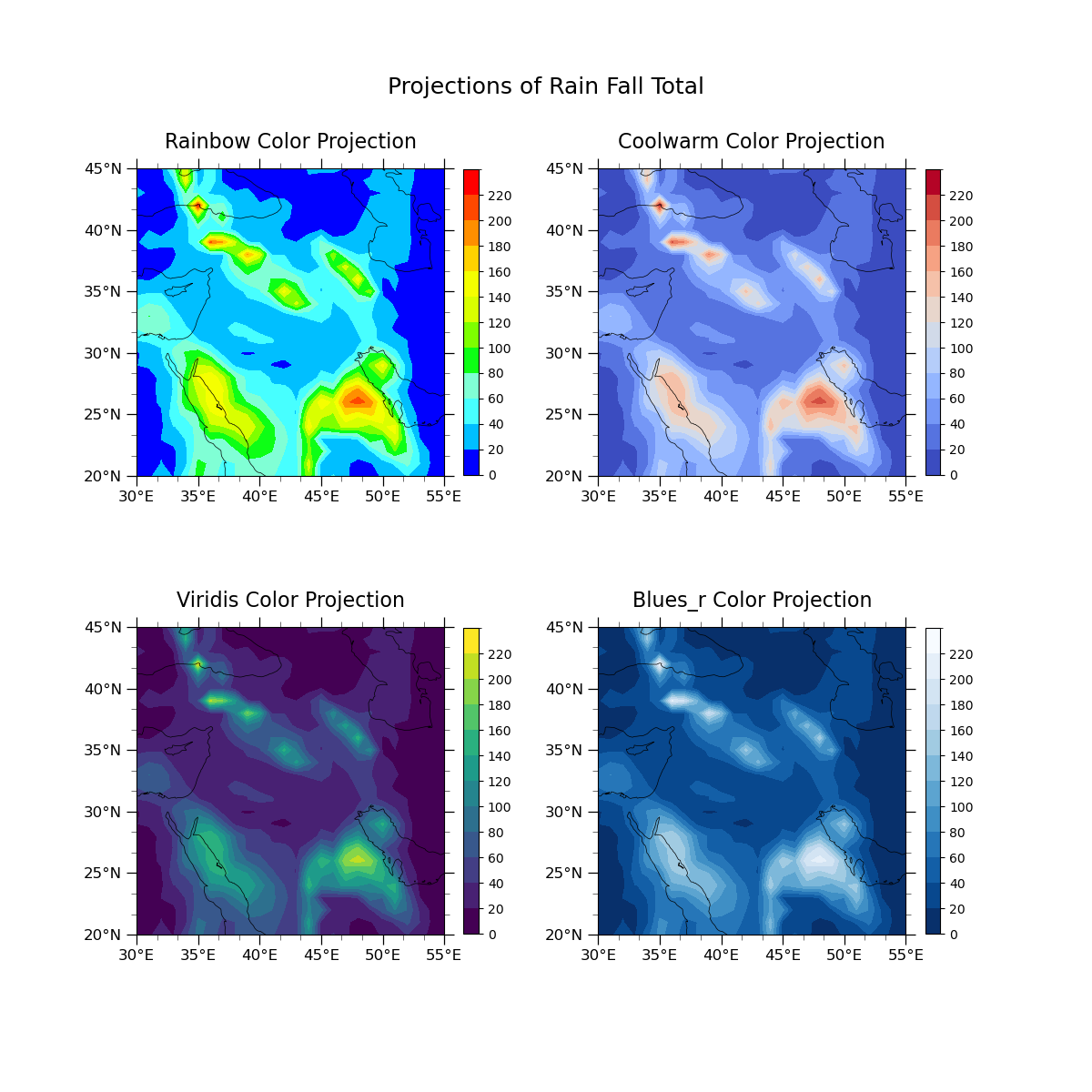# CB_Rain.py#

This script illustrates multiple color schemes for color maps which will allow for those impacted by color blindness to see visualizations. Using rainbow color schemes is also a poor choice in color scheme for images that may be transferred to a black and white scale for printing. This code addresses a handful of options to use in place of rainbow color schemes for use in the matplotlib.pyplot library.

More color schemes can be found here:
See following URL to see the reproduced plot & script from the GeoCAT examples gallery:
• Link to be produced when PR is merged

Figure 1.
• The rainbow color scheme is problematic due to the lack of a natural perceived ordering of colors, perceptual changes in the colors (ex: yellow and green blend together easily), and is sensitive to deficiencies in vision

Figure 2.
• The coolwarm diverging scheme should be used when both high and low values are interesting. However, be careful using this scheme if the projection will be printed to black and white.

Figure 3.
• This is an example of a less distinct contrasting color gradient. This choice in color scheme would be a good choice for printing in black and white but may create some challenges for individuals who experience blue-green colorblindness.

Figure 4.
• This plot shows how a singular color like blue can be incredibly useful for plotting this type of data. This color scheme will work well for color blind impacted individuals and is black and white print friendly.

Import packages:

```import cartopy.crs as ccrs
import cartopy.feature as cfeature
import matplotlib.pyplot as plt
import numpy as np
import xarray as xr

import geocat.datafiles as gdf
import cmaps
import geocat.viz as gv
```

```# Open a netCDF data file using xarray default engine and load the data into xarrays
ds = xr.open_dataset(gdf.get("netcdf_files/pre.8912.mon.nc"))

# Extract a slice of the data
t = ds.pre[0, :]
```

Plot:

```fig = plt.figure(figsize=(12, 12))

def Plot(color, row, col, pos, title):

# Generate axes, using Cartopy, drawing coastlines, and adding features
projection = ccrs.PlateCarree()
ax1 = plt.subplot(row, col, pos, projection=projection)
ax1.coastlines(linewidths=0.5)

# Import an NCL colormap
newcmp = color

# Contourf-plot data
pre = t.plot.contourf(ax=ax1,
transform=projection,
levels=14,
vmin=0,
vmax=240,
cmap=newcmp,

cbar_ticks = np.arange(0, 240, 20)
cbar = plt.colorbar(pre,
orientation='vertical',
shrink=0.8,
extendrect=True,
ticks=cbar_ticks)

cbar.ax.tick_params(labelsize=10)

# Use geocat.viz.util convenience function to set axes parameters without calling several matplotlib functions
# Set axes limits, and tick values
gv.set_axes_limits_and_ticks(ax1,
xlim=(30, 55),
ylim=(20, 45),
xticks=np.linspace(30, 55, 6),
yticks=np.linspace(20, 45, 6))

# Use geocat.viz.util convenience function to make plots look like NCL plots by using latitude, longitude tick labels

# Use geocat.viz.util convenience function to add minor and major tick lines

# Use geocat.viz.util convenience function to set titles and labels without calling several matplotlib functions
gv.set_titles_and_labels(ax1,
maintitle=title,
maintitlefontsize=16,
righttitlefontsize=14,
xlabel="",
ylabel="")

#Plot first color map
Plot(cmaps.BlAqGrYeOrRe, 2, 2, 1, "Rainbow Color Projection")

#plot second color map
Plot('coolwarm', 2, 2, 2, "Coolwarm Color Projection")

#plot third color map
Plot('viridis', 2, 2, 3, "Viridis Color Projection")

#Plot fourth color map
Plot('Blues_r', 2, 2, 4, "Blues_r Color Projection")

fig.suptitle("Projections of Rain Fall Total", x=.5, y=.93, fontsize=18)
``````Text(0.5, 0.93, 'Projections of Rain Fall Total')
```

Total running time of the script: ( 0 minutes 2.703 seconds)

Gallery generated by Sphinx-Gallery